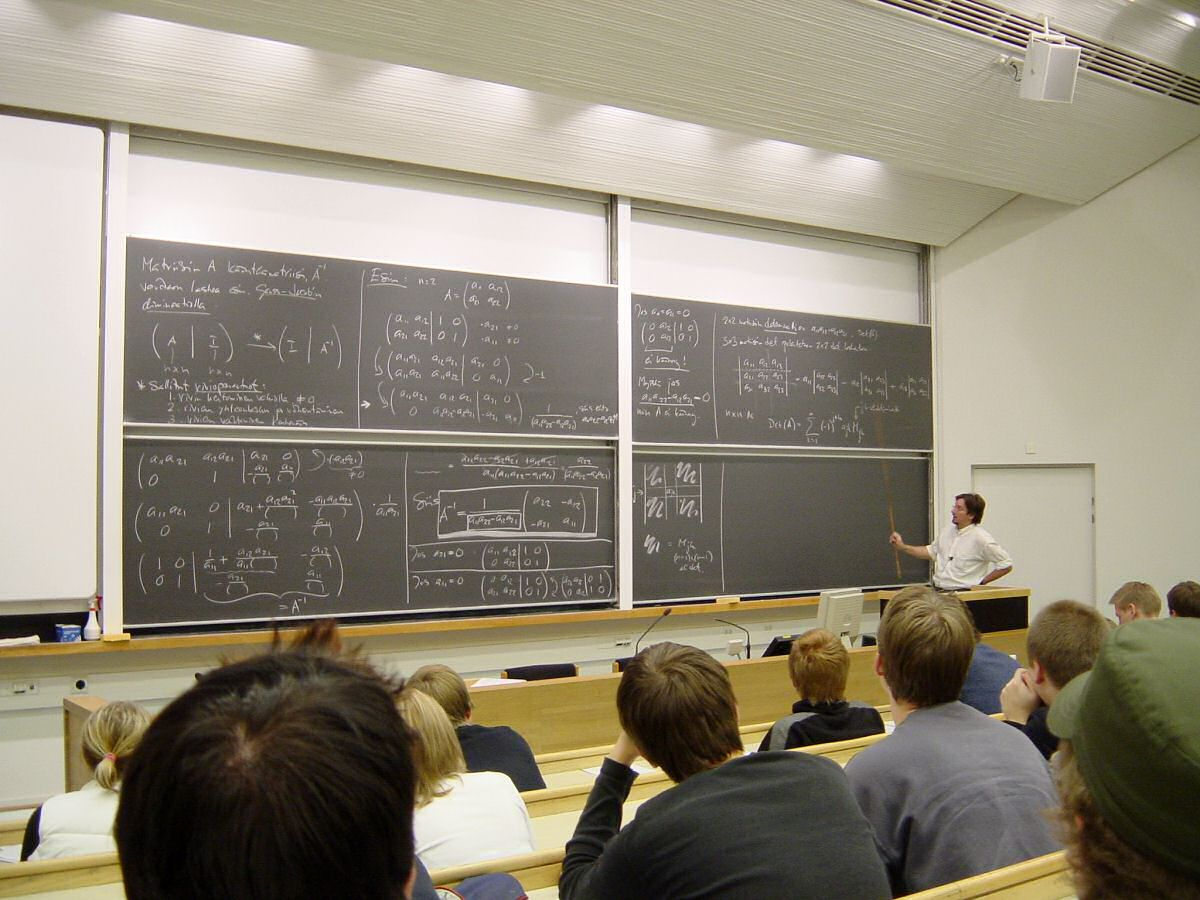Explore

Browse thousands of resources, organized by 90+ programs.

### Explore# Word Problem Strategies: Mind the UnitsImage fromAshish August 18, 2014

Studying for a math and struggling with word problems? Break it down and look at the units.

### Noodle Courses

Article continues here

If you're having trouble with word problems, one trick is to look closely at the units, as word problems that involve physical quantities always deal with them. Units are an important part of a correct answer, and they can even give you clues. Units come in two kinds, fundamental and compound. Fundamental units measure basic physical quantities, while compound units are made from a combination of fundamental units.

Fundamental units measure quantities such as distance, mass, and time. Compound units are units such as speed, which are combinations of these. Examples of units of all these types are listed below.

• Inch
• Foot
• Mile
• Meter
• Centimeter
• Kilometer

• Second
• Minute
• Hour
• Day
• Week
• Month
• Year

• Pound
• Ton
• Gram
• Kilogram

# Compound Units

• Speed – distance/time
• Acceleration – distance/time, squared
• Area – distance, squared
• Volume – distance, cubed

The science of working with units is called dimensional analysis. Units multiply and divide much like algebraic quantities. For example, to get a speed, we divide a distance by a time (miles per hour), and to get a distance, we multiply a speed by a time (driving 40 miles per hour for 2 hours, you go 80 miles). If you take note of the units required by the answer, that can give you a clue about how to combine the elements in the problem to get the desired result.

Example: If Marvin can process 5 batches per hour, and each batch contains 20 cases, how long will it take him to process 800 cases?*

Solution: We have 5 batches per hour and 20 cases per batch, which we can write as 5 batches/hour and 20 cases/batch. Thus 800 cases / 20 cases/batch = 40 batches, and 40 batches / 5 batches/hour = 8 hours.

Share